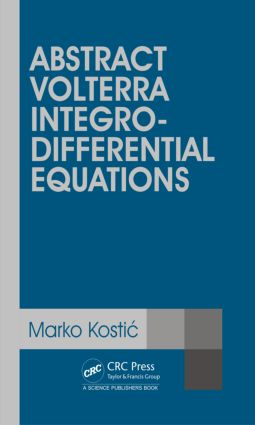# Abstract Volterra Integro-Differential Equations

## 1st Edition

CRC Press

484 pages

Paperback: 9780367377670
pub: 2019-07-31
SAVE ~\$14.99
Available for pre-order
\$74.95
\$59.96
x
Hardback: 9781482254303
pub: 2015-05-06
SAVE ~\$33.00
\$165.00
\$132.00
x
eBook (VitalSource) : 9780429162503
pub: 2015-05-06
from \$28.98

FREE Standard Shipping!

### Description

The theory of linear Volterra integro-differential equations has been developing rapidly in the last three decades. This book provides an easy to read concise introduction to the theory of ill-posed abstract Volterra integro-differential equations. A major part of the research is devoted to the study of various types of abstract (multi-term) fractional differential equations with Caputo fractional derivatives, primarily from their invaluable importance in modeling of various phenomena appearing in physics, chemistry, engineering, biology and many other sciences. The book also contributes to the theories of abstract first and second order differential equations, as well as to the theories of higher order abstract differential equations and incomplete abstract Cauchy problems, which can be viewed as parts of the theory of abstract Volterra integro-differential equations only in its broad sense. The operators examined in our analyses need not be densely defined and may have empty resolvent set.

Divided into three chapters, the book is a logical continuation of some previously published monographs in the field of ill-posed abstract Cauchy problems. It is not written as a traditional text, but rather as a guidebook suitable as an introduction for advanced graduate students in mathematics or engineering science, researchers in abstract partial differential equations and experts from other areas. Most of the subject matter is intended to be accessible to readers whose backgrounds include functions of one complex variable, integration theory and the basic theory of locally convex spaces. An important feature of this book as compared to other monographs and papers on abstract Volterra integro-differential equations is, undoubtedly, the consideration of solutions, and their hypercyclic properties, in locally convex spaces. Each chapter is further divided in sections and subsections and, with the exception of the introductory one, contains a plenty of exam

PREFACE. NOTATION. INTRODUCTION. PRELIMINARIES. Vector-valued functions, closed operators and integration in sequentially complete locally convex spaces. Laplace transform in sequentially complete locally convex spaces. Operators of fractional differentiation, Mittag-Leffler and Wright functions. (a; k)-REGULARIZED C-RESOLVENT FAMILIES IN LOCALLY CONVEX SPACES. Definition and main structural properties of (a; k)-regularized C-resolvent families. Wellposedness of related abstract Cauchy problems. Convoluted C-semigroups and convoluted C-cosine functions in locally convex spaces. Differential and analytical properties of (a; k)-regularized C-resolvent families. Systems of abstract time-fractional equations.q-Exponentially equicontinuous (a; k)-regularized C-resolvent families. Abstract differential operators generating fractional resolvent families. Perturbation theory for abstract Volterra equations. Bounded perturbation theorems. Unbounded perturbation theorems. Time-dependent perturbations of abstract Volterra equations. Approximation and convergence of (a; k)-regularized C-resolvent families. (a; k)-Regularized (C1;C2)-existence and uniqueness families. Complex powers of (a; b;C)-nonnegative operators and fractional resolvent families generated by them. Complex powers of a C-sectorial operator A satisfying 0 2 (□C(A))□. The Balakrishnan operators. Complex powers of almost C-nonnegative operators. The case m = ����1. Semigroups generated by fractional powers of almost C-sectorial operators. Fractional powers of (a; b;C)-nonnegative operators and semigroups generated by them. The existence and growth of mild solutions of operators generating fractionally integrated C-semigroups and cosine functions in locally convex spaces. Representation of powers. Abstract multi-term fractional differential equations. k-Regularized (C1;C2)-existence and uniqueness propagation families for (2). k-Regularized (C1;C2)-existence and uniqueness families for (2). Approximation and conv

Marko Kostic